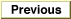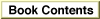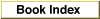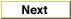# Legacy DocumentImportant: The information in this document is obsolete and should not be used for new development.

Inside Macintosh: QuickDraw GX Environment and Utilities /
Chapter 8 - QuickDraw GX Mathematics / QuickDraw GX Mathematics Reference
Mathematical Functions / Fixed-Point Operations

### MultiplyDivide

You can use the `MultiplyDivide` function to multiply two numbers and divide by a third number.

```long MultiplyDivide (long multiplicand, long multiplier,
long divisor);
```
`multiplicand`
The number to be multiplied by the multiplier.
`multiplier`

The number by which the multiplicand is multiplied.
`divisor`
The number by which the product is divided.
function result
The quotient of the product of two numbers and the divisor.
##### DESCRIPTION
The `MultiplyDivide` function calculates the quotient of the product of two numbers (parameters `multiplicand` and `multiplier`) and a divisor.

The function uses a 64-bit intermediate result to maintain accuracy and to prevent premature overflow. The parameters do not need to all be the same fixed-point format. The operation has a bias of 0 bits; if the divisor is the same format as the multiplier, the result is the same format as the multiplicand. If the divisor is the same format as the multiplicand, the result is in the same format as the multiplier.

##### SPECIAL CONSIDERATIONS
In the case of overflow, `MultiplyDivide` pins its result to either the `gxPositiveInfinity` or `gxNegativeInfinity` constant.